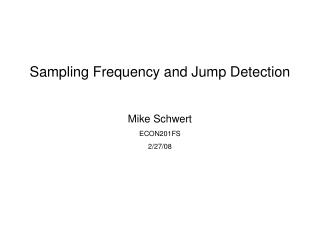Download PresentationSampling Frequency and Jump Detection Mike Schwert ECON201FS 2/27/08

# Sampling Frequency and Jump Detection Mike Schwert ECON201FS 2/27/08 - PowerPoint PPT PresentationDownload Presentation## Sampling Frequency and Jump Detection Mike Schwert ECON201FS 2/27/08

- - - - - - - - - - - - - - - - - - - - - - - - - - - E N D - - - - - - - - - - - - - - - - - - - - - - - - - - -
##### Presentation Transcript

1. Sampling Frequency and Jump Detection Mike Schwert ECON201FS 2/27/08

2. This Week’s Approach • Last time: • Counted jump days at various sampling frequencies • Volatility signature plots for RV and BV • This week: • Semivariance calculations for GE price data • Volatility signature plots for RJ, RS- and RS+ • Counted common jump days between different sampling frequencies • Correlation matrices for ZQP-max statistics at different sampling frequencies

3. Realized Semivariance • Introduced by Barndorff-Nielsen, Kinnebrock, and Shephard • Separates positive and negative components of realized variance Summary Statistics – GE Price Data, 5-minute sampling frequency

4. Volatility Signature Plots • Introduced by Andersen, Bollerslev, Diebold, and Labys (1999). • Calculate RJ, RS-, RS+ at 1, 2, …, 30 minute sampling frequencies • Plot relationship between sampling frequency and mean RJ, mean RS • RJ and RS are higher for high-frequency samples because returns are distorted by microstructure noise such as bid-ask bounce • Must be wary of using too low of a sampling frequency, as sampling variation will affect volatility calculations

5. Volatility Signature Plot – Relative Jump

6. Volatility Signature Plot – Negative Semivariance

7. Volatility Signature Plot – Positive Semivariance

8. Contingency and Correlation Matrices • Calculated ZQP-max statistics and counted jump days for GE, ExxonMobil, AT&T, and S&P 500 at 5, 10, 15 and 20 minute sampling frequencies • Counted common jump days between each sampling frequency and organized in contingency matrices • Surprisingly few common jump days exist between sampling frequencies • Calculation of jump days seems to depend a great deal on sampling choices • ZQP-max statistics are relatively uncorrelated between sampling frequencies • GE data minute-by-minute for 1997 – 2007 (2670 days) • ExxonMobil data minute-by-minute for 1999 – 2008 (2026 days) • AT&T data minute-by-minute for 1997 – 2008 (2680 days) • S&P data every 5 minutes, 1985 – 2007 (5545 days, excluding short days)

9. Contingency Tables GE S&P 500 Exxon Mobil AT&T

10. Correlation Matrices - Z-Statistics GE S&P 500 – N/A Exxon Mobil AT&T

11. Possible Extensions • Try other jump test statistics to see if one outperforms the others in detecting jumps consistently between sampling frequencies • Regress z-statistics on changes in daily volume to see if days with high volume correspond to jump days, common jump days between samples • Other ways to formally analyze effects of sampling frequency on jump detection? • Any way to separate negative jumps from positive jumps? • Effect of sampling frequency on volatility forecasts?